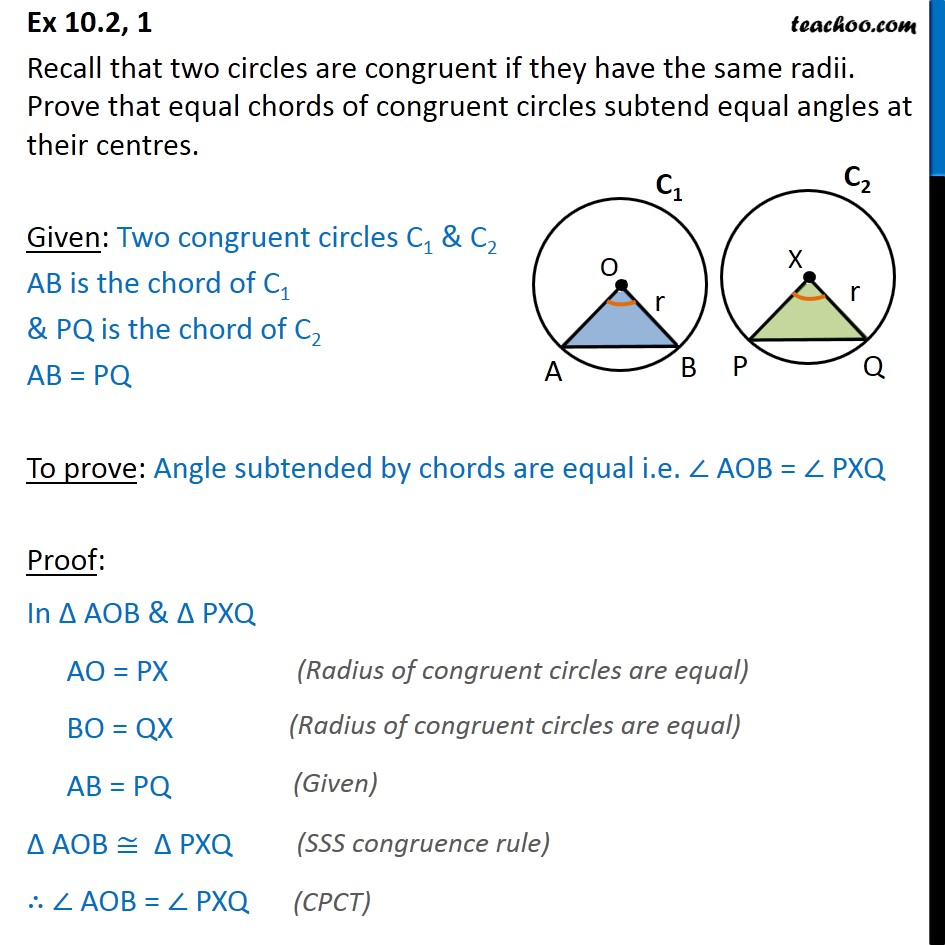1. Chapter 10 Class 9 Circles (Term 2)
2. Serial order wise
3. Ex 10.2

Transcript

Ex 10.2, 1 Recall that two circles are congruent if they have the same radii. Prove that equal chords of congruent circles subtend equal angles at their centres. Given: Two congruent circles C1 & C2 AB is the chord of C1 & PQ is the chord of C2 AB = PQ To prove: Angle subtended by chords are equal i.e. AOB = PXQ Proof: In AOB & PXQ AO = PX BO = QX AB = PQ AOB PXQ AOB = PXQ